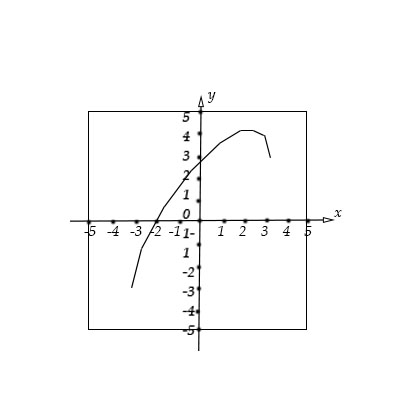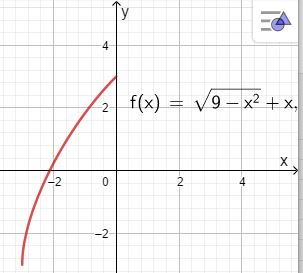# Given the graph of f(x)=\sqrt {9-x^2}+x locate he absolute extreme (if they exist) on the...

## Question:

Given the graph of {eq}f(x)=\sqrt {9-x^2}+x {/eq} locate he absolute extreme (if they exist) on the interval {eq}[-3,0] {/eq}## Absolute Extremes:

The absolute extremes of a function are the highest (Maximum) and lowest (Minimum) values that the function can have in an interval or in its entire domain.

We have the function

{eq}f(x)=\sqrt {9-x^2}+x {/eq}

Differentiating the function

{eq}f'(x)=-{\frac {x}{\sqrt {-{x}^{2}+9}}}+1 \\ {/eq}

{eq}f'(x)=0 {/eq} when {eq}x= \frac{3}{2} \sqrt{2} \\ {/eq} and {eq}f'(x)=DNE {/eq} when {eq}x= 3 \\ x=-3 \\ {/eq}

Graph the function on the interval {eq}[-3, 0] {/eq}Therefore,

Absolute extremes are:

Absolute maximum:

{eq}(0, 3) {/eq}

Absolute minimum:

{eq}(-3, -3) {/eq}